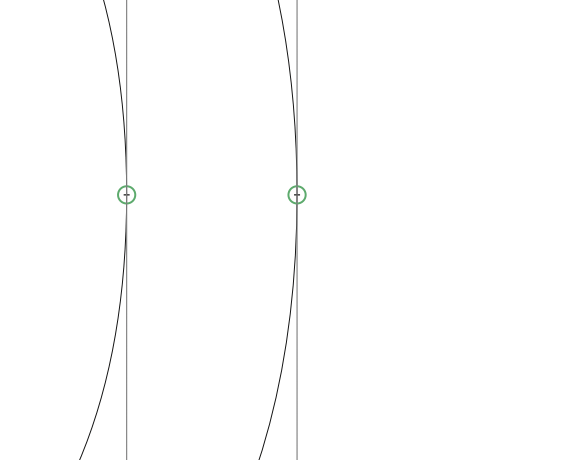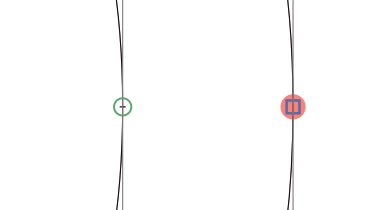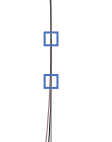# Split node script anyone?

Does anyone maybe have a script to split a node ? Like for example a curve point into two cornerpoints.

Just so that we are on the same page, can you share a screenshot of what you intend, before and after?

In FL there used to be a command to “divide” or split nodes into two.

First pic: status quo
Second: “split node”
Third: Goal. That is, I want to add a line in between. (in this sample).``````node = Layer.selection
print node
if isinstance(node, GSNode):
if node.type == LINE or node.type == CURVE:
node.smooth = False
newNode = node.copy()
newNode.type = LINE
path = node.parent
path.nodes.insert(node.index + 1, newNode)
else:
print "select one node"
``````
4 Likes

Cool ! Thanks @GeorgSeifert

maybe an updated version of this for GL3 @GeorgSeifert?Just add brackets around the print statement:

``````node = Layer.selection

print(node)
if isinstance(node, GSNode):
if node.type == LINE or node.type == CURVE:
node.smooth = False
newNode = node.copy()
newNode.type = LINE
path = node.parent
path.nodes.insert(node.index + 1, newNode)
else:
print("select one node")
``````